# MASS: Masked Sequence to Sequence Pre-training for Language Generation

## MASS

### Sequence to Sequence Learning

\begin{aligned} L(\theta ;(\mathcal{X}, \mathcal{Y}))&=\sum_{(x, y) \in(\mathcal{X}, \mathcal{Y})} \log P(y \mid x ; \theta)\\ &=\sum_{(x, y) \in(\mathcal{X}, \mathcal{Y})} \log \prod_{t=1}^{n} P\left(y_{t} \mid y_{<t}, x ; \theta\right) \end{aligned}

### Masked Sequence to Sequence Pre - Training

MASS(MAsked Sequence to Sequence Pre - training for Language Generation)中, 不再采用句子对的方法训练模型, 而是采用去噪编码器的方式训练模型.

\begin{aligned} L(\theta ; \mathcal{X}) &=\frac{1}{|\mathcal{X}|} \Sigma_{x \in \mathcal{X}} \log P\left(x^{u: v} \mid x^{\backslash u: v} ; \theta\right) \\ &=\frac{1}{|\mathcal{X}|} \Sigma_{x \in \mathcal{X}} \log \prod_{t=u}^{v} P\left(x_{t}^{u: v} \mid x_{<t}^{u: v}, x^{\backslash u: v} ; \theta\right) \end{aligned}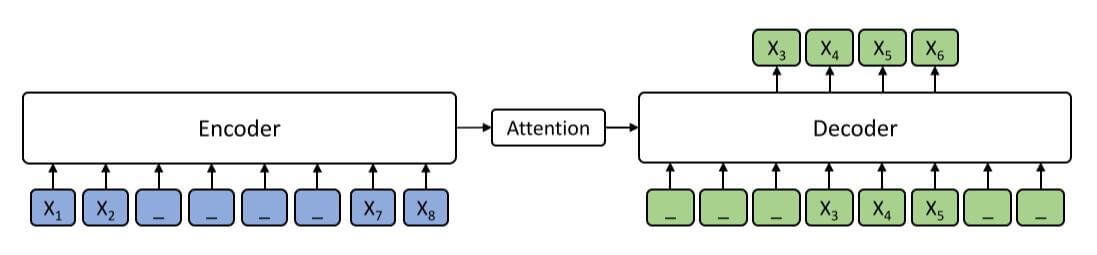• BERT: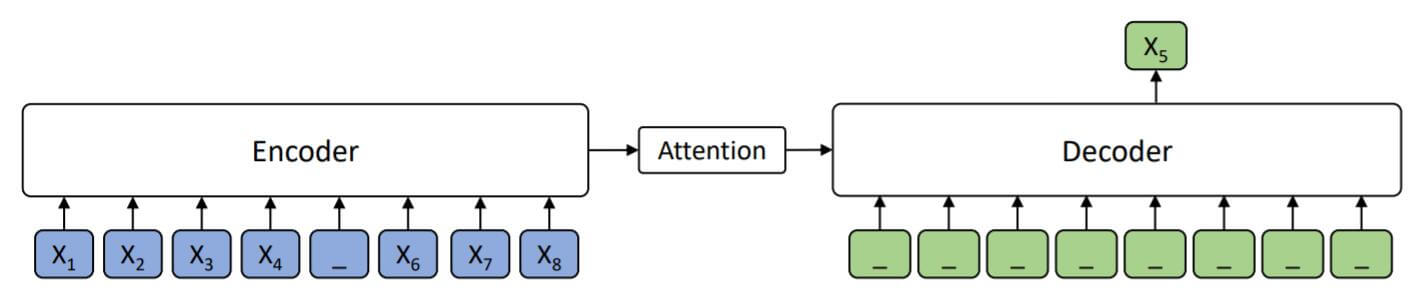当$k=1$ 时, Decoder没有输入任何额外信息, 单纯利用Encoder的信息预测出被Mask掉的Token. 从双向利用上下文的角度来说, BERT是MASS的一种特殊情况.

• GPT: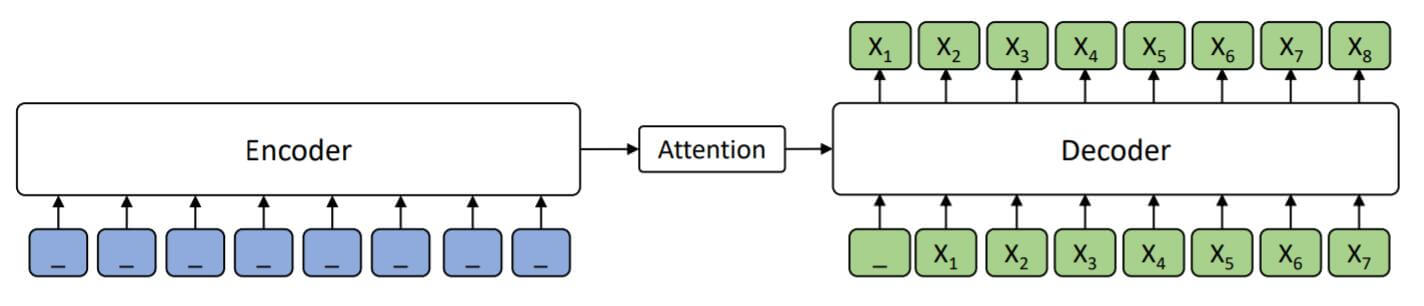当$k=m$ 时, MASS直接就变成了自回归式的生成模型, 需要预测所有的Token. Encoder没有给Decoder任何信息.

• MASS:

MASS便是介于BERT和GPT二者之间的, $k\in(1, m)$.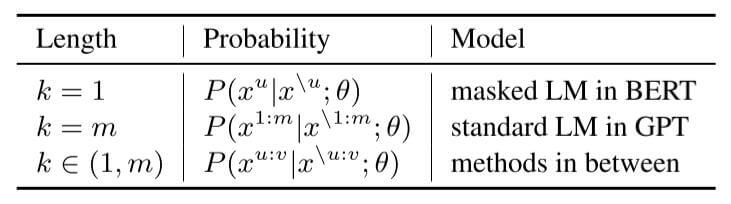### Discussions

• Encoder和Decoder联合训练. 在其他语言模型或BERT中都只是单独训练Encoder或Decoder.

这点是针对预训练来说的, MASS将问题转化为Seq2Seq后, 当然可以做到对于任何问题, 都用联合训练好的Encoder和Decoder解决.

• 使Decoder能从Encoder获取更多的信息, 而不是获取Decoder自身生成的信息.

## Experiments

### Neural Meachine Translation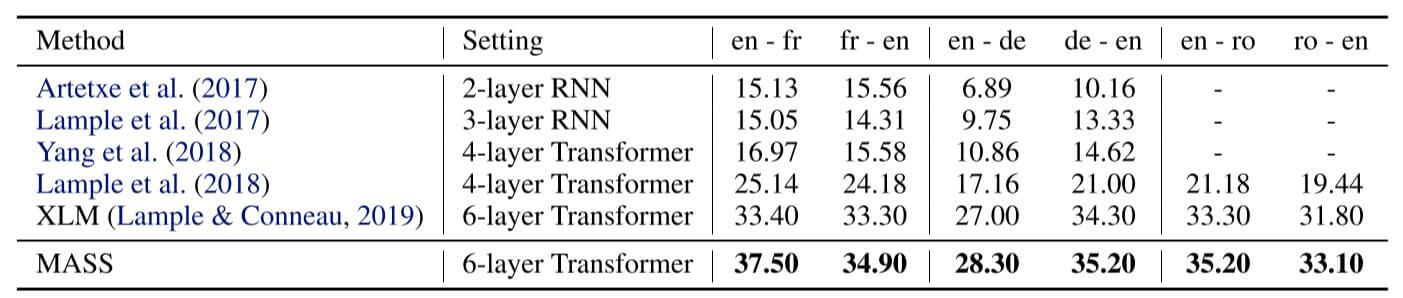MASS在无监督机器翻译上表现还可以, 除了英语翻译到法语, 剩下的数据集基本上涨了一个点.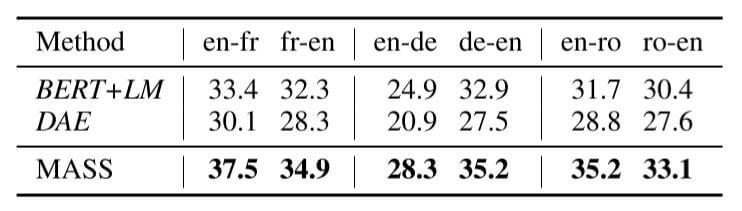MASS比其他的预训练方法平均涨了3个点左右.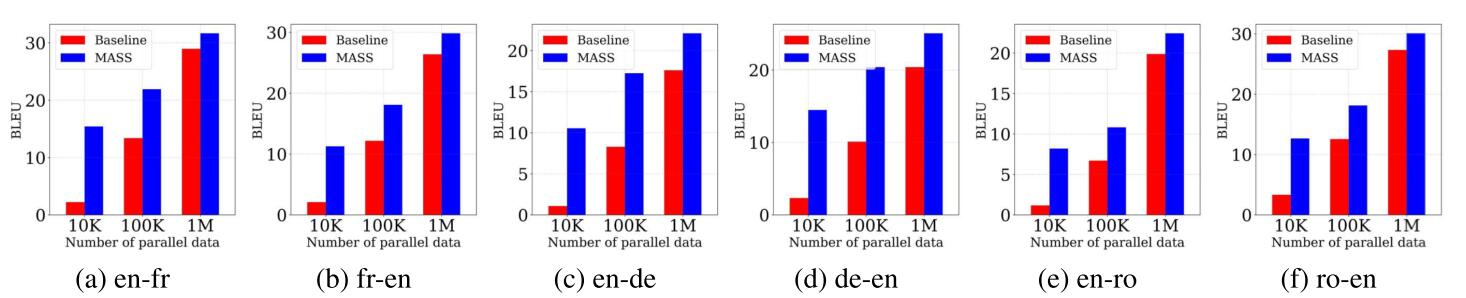### Text Summarization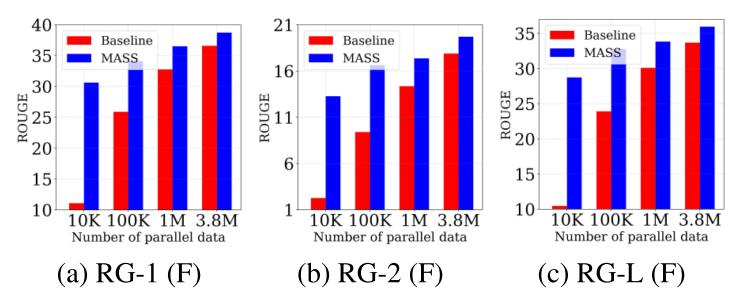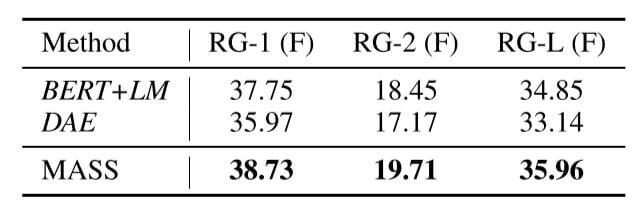MASS结果有些许提升.

### Conversational Response Generation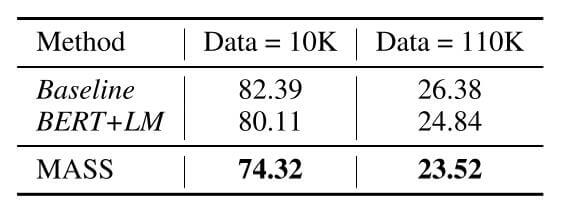### Analysis of MASS

#### Study of Different k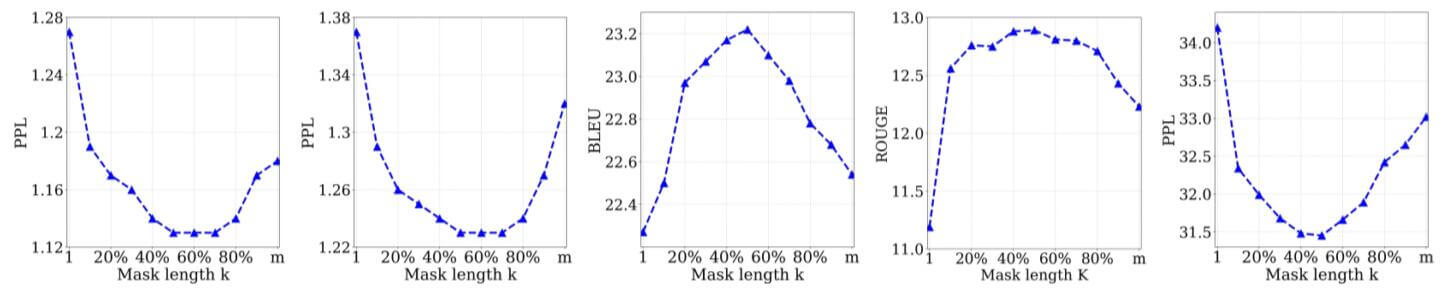#### Ablation Study of MASS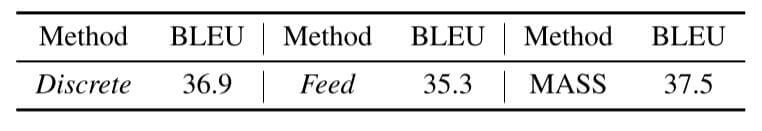MASS的这两个改动确实有性能提升, 但似乎没有特别大的变化.

## Summary

MASS与BART属于相同类型的模型, 二者处理思路都是重新把BERT拉回到Seq2Seq框架下做生成任务. 这二者的侧重点不同, MASS更侧重对Decoder的改进, 而BART更侧重对加噪方法做出调整.

上一篇UniLM: Unified Language Model Pre-training for Natural Language Understanding and Generation

2021-06-18SpanBERT: Improving Pre-training by Representing and Predicting Spans

2021-05-13
目录